Premium

# 4.OA.A.2 Worksheets, Workbooks, Lesson Plans, and Games

#### CCSS.maths.CONTENT.4.OA.A.2

:
"Multiply or divide to solve word problems involving multiplicative comparison, e.g., by using drawings and equations with a symbol for the unknown number to represent the problem, distinguishing multiplicative comparison from additive comparison."

These worksheets, games, and lesson plans can help students practise this Common Core State Standards skill.

## Worksheets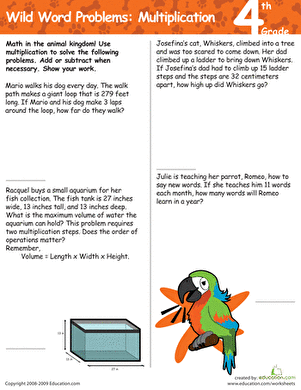Wild Word Problems: Multiplication
Worksheet
Wild Word Problems: Multiplication
These multi-digit multiplication word problems draw inspiration from the animal kingdom.
Year 5
Maths
Worksheet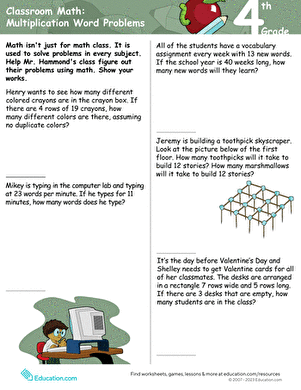Classroom maths: Multiplication Word Problems
Worksheet
Classroom maths: Multiplication Word Problems
Your fourth grader will be challenged to complete five word problems using multiplication with two-digit numbers.
Year 5
Maths
Worksheet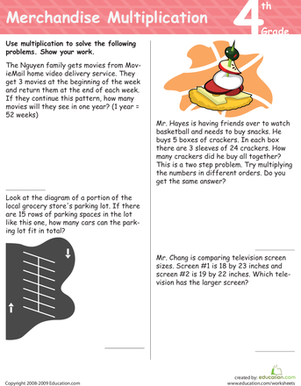Merchandise Multiplication
Worksheet
Merchandise Multiplication
These word problems will get your fourth grader multiplying using real-life scenarios!
Year 5
Maths
Worksheet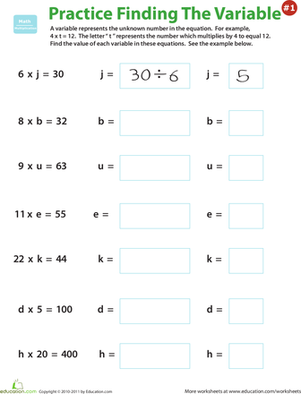Practise Finding the Variable #1
Worksheet
Practise Finding the Variable #1
Give your fourth grader practise with multiplication equations that include variables -- a good excuse to practise division!
Year 5
Worksheet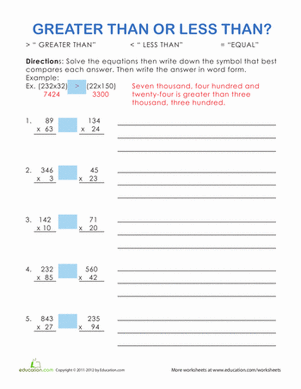Greater Than or Less Than? #1
Worksheet
Greater Than or Less Than? #1
Do you remember your greater than or less than signs? Give your student some great practise solving long multiplication with this maths worksheet.
Year 5
Maths
Worksheet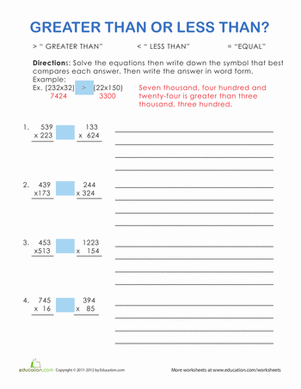Greater Than or Less Than? #2
Worksheet
Greater Than or Less Than? #2
Don't mix up your greater or less than signs! Give your student some great practise doing long multiplication with this maths worksheet.
Year 5
Maths
Worksheet

## Lesson PlansFood Fractions: What are the Odds?
Lesson plan
Food Fractions: What are the Odds?
Students are sure to love this sweet lesson that teaches them fractions and probability with candy!
Year 5
Maths
Lesson plan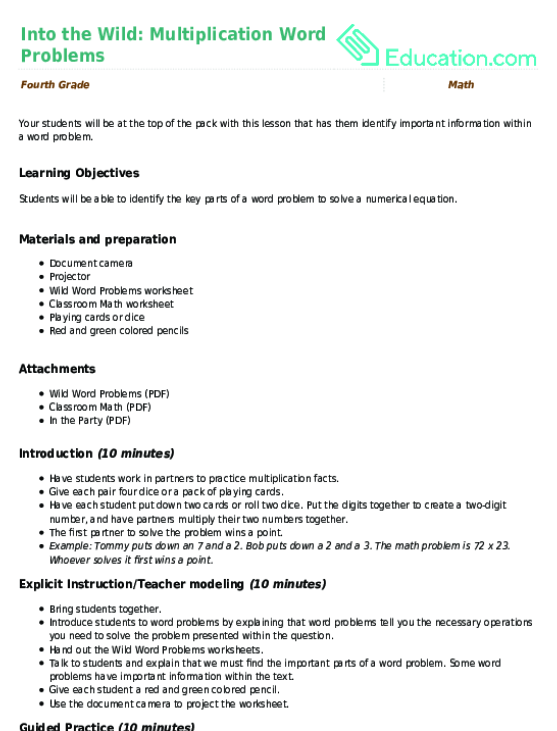Into the Wild: Multiplication Word Problems
Lesson plan
Into the Wild: Multiplication Word Problems
Your students will be at the top of the pack with this lesson that has them identify important information within a word problem.
Year 5
Maths
Lesson planAnimal Kingdom Comparisons
Lesson plan
Animal Kingdom Comparisons
How much longer is a blue whale than an orca? Teach your students to use bar models and number sentences with variables as they solve these wild word problems!
Year 5
Maths
Lesson plan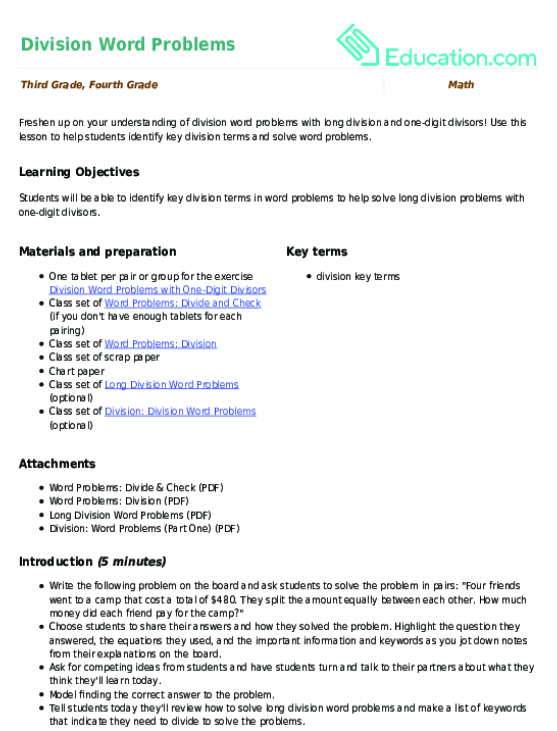Division Word Problems
Lesson plan
Division Word Problems
Freshen up on your understanding of division word problems with long division and one-digit divisors! Use this lesson to help students identify key division terms and solve word problems.
Year 4
Maths
Lesson planDeciphering Division Word Problems
Lesson plan
Deciphering Division Word Problems
Word problems present an additional challenge for English learners. This division lesson gives them a concrete strategy to decipher word problems. Teach it on its own or prior to teaching Division Word Problems.
Year 5
Maths
Lesson plan

## Workbooks

No workbooks found for this common core node.

## Games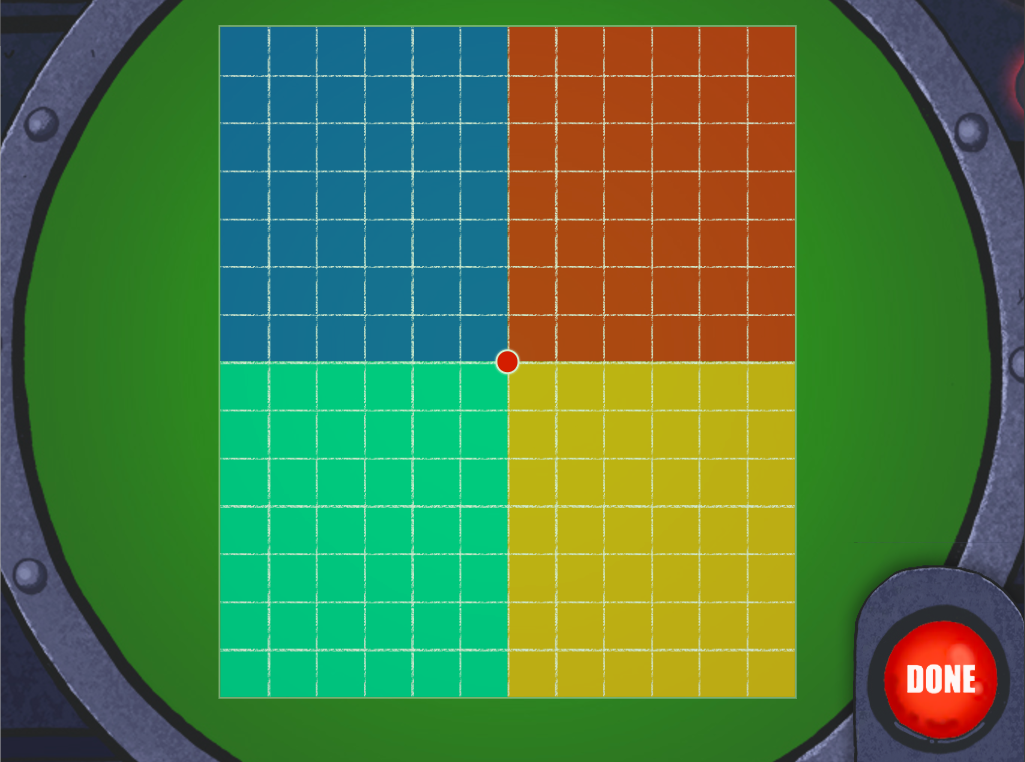Radar Multi-Digit Arrays
Game
Radar Multi-Digit Arrays
Kids find the products of arrays to help Muggo's fleet course correct in this radar game.
Year 5
Maths
Game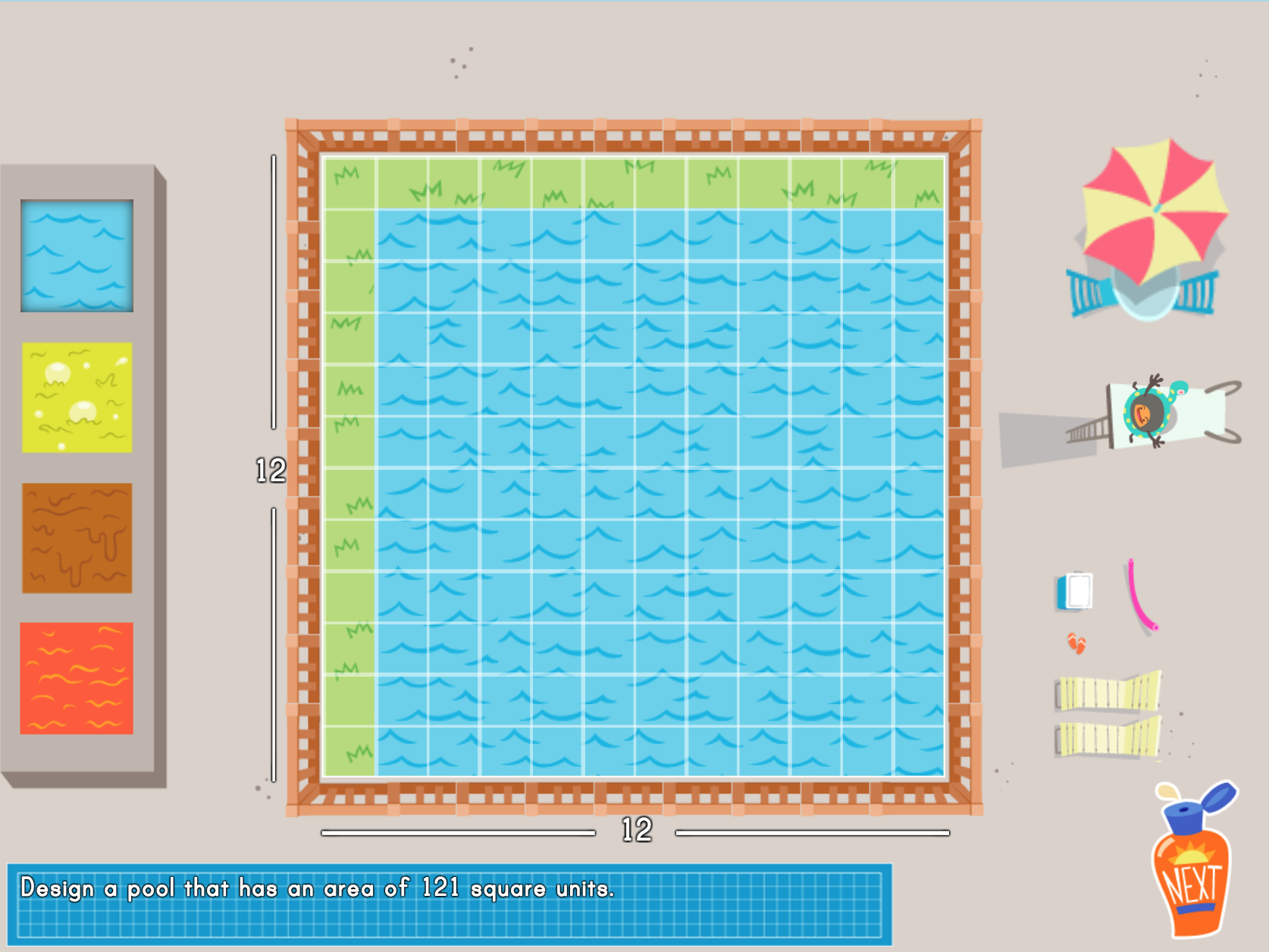Splash maths Multiplication Word Problem Game
Game
Splash maths Multiplication Word Problem Game
Splish, splash! Kids design pools with specific areas and use multiple problem-solving strategies in this game.
Year 5
Maths
Game

## Exercises

No exercises found for this common core node.

### Add to collection

Create new collection

0

### New Collection>

0Items

What could we do to improve Education.com?

Please note: Use the Contact Us link at the bottom of our website for account-specific questions or issues.

What would make you love Education.com?